# AP Chemistry : Polyatomic Ions and Functional Groups

## Example Questions

### Example Question #31 : Compounds And Molecules

What is the oxidation state of manganese (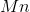) in the polyatomic permanganate anion (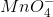)?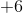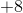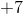Explanation:

When assigning oxidation states to elements of a given compound, non-transition metal elements are assigned specific oxidation states corresponding to their group number and valence relative to a complete octet.

Group 1 elements have 1 valence electron and an oxidation state of +1.

Group 2 elements have 2 valence electrons and an oxidation state of +2.

Group 8 elements (the noble gases) have a complete octet, thus their assigned oxidation state is 0.

Group 7 elements (halogens) have 7 valence electrons and an oxidation state of -1.

Group 6 elements such as oxygen have 6 valence electrons and an oxidation state of -2.

Permanganate has 4 oxygen atoms and an overall charge of -1. The oxidation state of theatom may be found by first calculating the combined oxidation state of the oxygen atoms: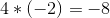and finding the difference between their combined oxidation state and the overall charge of the ion (-1):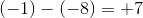### Example Question #32 : Compounds And Molecules

According to VSEPR theory, for the ammonium ion (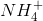), the electron-pair geometry is __________ and the molecular geometry is __________.

tetrahedral . . . trigonal pyramidal

trigonal pyramidal . . . tetrahedral

tetrahedral . . . tetrahedral

trigonal pyramidal . . . trigonal pyramidal

tetrahedral . . . tetrahedral

Explanation:

Both the electron-pair and molecular geometries of ammonium are tetrahedral, as opposed to ammonia (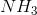), in which the electron-pair geometry is tetrahedral, but the molecular geometry is trigonal pyramidal. As evidenced below:

Ammonia: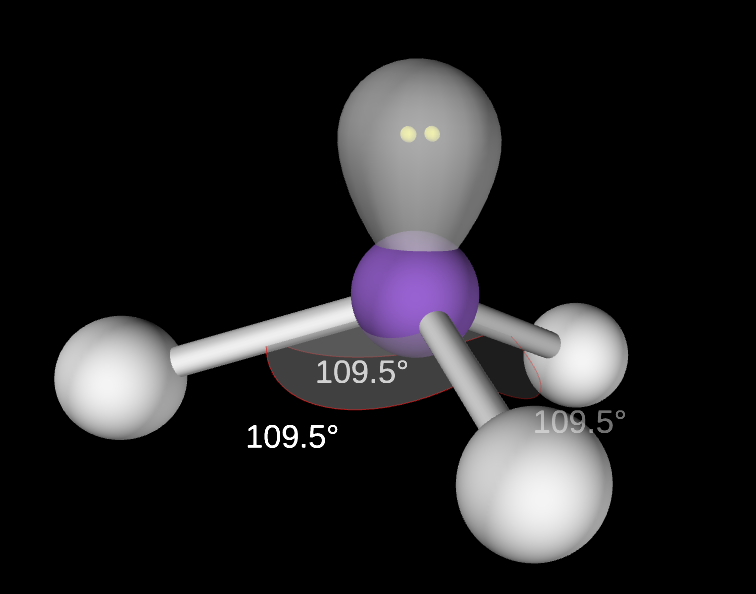Note that the difference in geometry arises in the presence of the lone pair on nitrogen in ammonia. The lone pair only contributes to molecular geometry, but does not contribute to electron-pair geometry. Bonding electrons, however, contribute to both electron and molecular geometries.

Ammonium: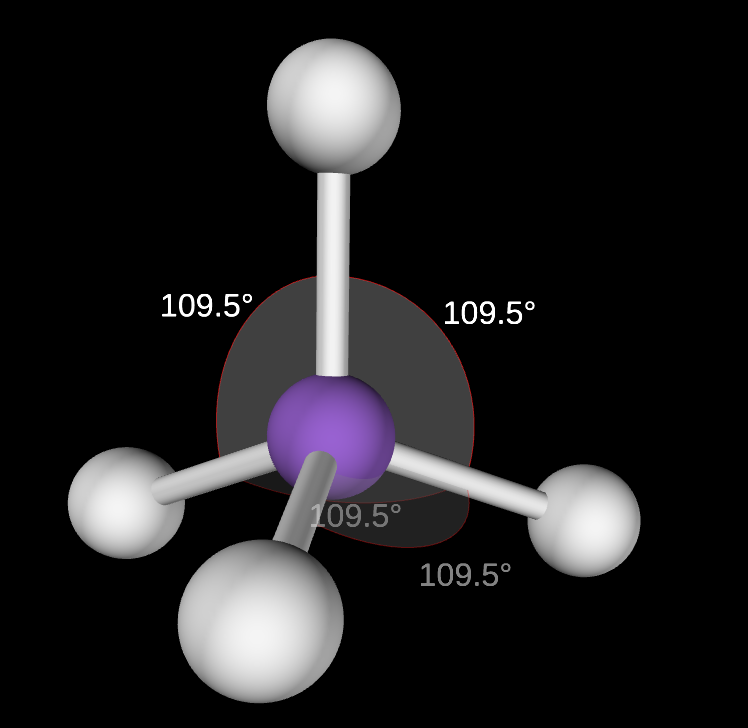In ammonium, the lone pair seen in ammonia is shared in a bond with an additional hydrogen atom.

### Example Question #33 : Compounds And Molecules

What are the mass percents of C, H, and O, respectively, in a molecule of glucose monomer (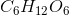)?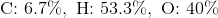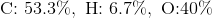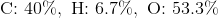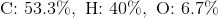Explanation:

On average, the molar masses of carbon, hydrogen, and oxygen are approximately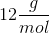,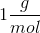, and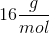, respectively.

The molar mass of a molecule of glucose is: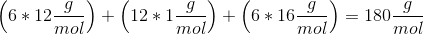Each term added in the previous step is the mass of each element present in a mole of glucose, so the mass percent of its components are found by dividing their respective contributions to the molar mass by the molar mass: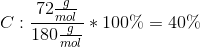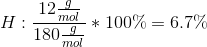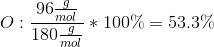### Example Question #41 : Compounds And Molecules

What is the name for the following polyatomic ion: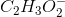(also written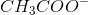)?

Carbon trihydrogen monocarbon dioxide

Acetate

Dicarbon trihydrogen dioxide

Dichromate

Oxalate(also written as) is the formula for the acetate ion. Most polyatomic ions tend to follow certain naming trends, but acetate is one of the ions that does not follow those naming trends, so memorize this one since it shows up frequently in chemistry.